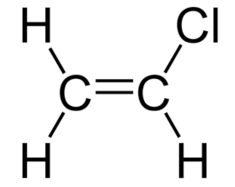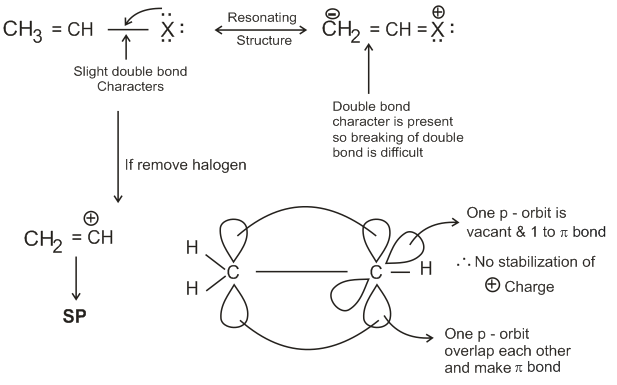# An 'Assertion' and a 'Reason' are given below. Choose the correct answer from the following options:Assertion (A): Vinyl halides do not undergo nucleophilic substitution easily.Reason (R): Even though the intermediate carbocation is stabilized by loosely held the cleavage is difficult because of strong bonding.Option 1)Both (A) and (R) are wrong statementsOption 2)Both (A) and (R) are the correct statement and (R) is the correct explanation of (A).  Option 3)Both (A) and (R) are correct statements but (R) isnot the correct explanation of (A).  Option 4)(A) is a correct statement but (R) wrong statement.

Vinayl Chloride -

Halogen present on double bonded carbon atom.

- whereinReactivity Order of alkyl halides -- whereinInhibition by steric hindrance SN2 reactions are particularly sensitive to steric factors, since they are greatly retarded by steric hindrance (crowding) at the site of reaction. In general, the order of reactivity of alkyl halides in SN2 reactions is: methyl  > 1> 2o> 3o

vinyl halides do not undergo a substitution reaction. It is a fact so, it is true statement

(R)Option 1)

Both (A) and (R) are wrong statements

Option 2)

Both (A) and (R) are the correct statement and (R) is the correct explanation of (A).

Option 3)

Both (A) and (R) are correct statements but (R) isnot the correct explanation of (A).

Option 4)

(A) is a correct statement but (R) wrong statement.

Vinayl Chloride -

Halogen present on double bonded carbon atom.

- whereinReactivity Order of alkyl halides -- whereinInhibition by steric hindrance SN2 reactions are particularly sensitive to steric factors, since they are greatly retarded by steric hindrance (crowding) at the site of reaction. In general, the order of reactivity of alkyl halides in SN2 reactions is: methyl  > 1> 2o> 3o

vinyl halides do not undergo a substitution reaction. It is a fact so, it is true statement

(R)Option 1)

Both (A) and (R) are wrong statements

Option 2)

Both (A) and (R) are the correct statement and (R) is the correct explanation of (A).

Option 3)

Both (A) and (R) are correct statements but (R) isnot the correct explanation of (A).

Option 4)

(A) is a correct statement but (R) wrong statement.